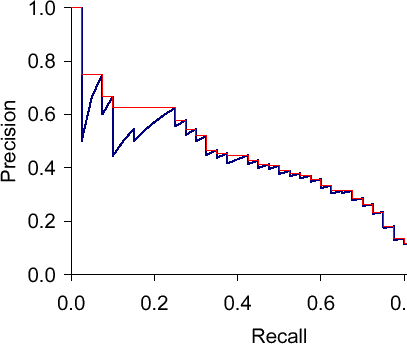# Precision-Recall-Curves and Mean Average Precision

Precision-recall curves are often used to evaluate ranked results of an information retrieval system (e.g., a search engine). The principle is easy, for every search result, check the precision and recall you have until now (precision/recall at k). If you plot this in a graph with recall on the x-axis and precision on the y-axis, you end up with something like this (blue line):The essential shape is always the same. Why? Let’s say we have looked at k results which corresponds to a point with a precision and a recall value. What can happen when we go to result k+1? The result can be correct, then recall will increase and precision as well – the curve goes up and right. Or it can go wrong, then recall stays the same and precision drops – the curve goes straight down.

The red line is the interpolated precision, meaning we define precision at some arbitrary level to be the maximum precision reached at any later recall level. Essentially, we flatten the "teeth" of the curve. The difference can be pretty big (see in the plot at recall around 0.2), we can even "skip" a tooth.

What would the curve look like for a perfect system? Meaning a system that only returns correct results? It would be 1.0 for every recall level. A system that never returns a correct result? 0 for every recall level.

What should the value be for precision at recall 0? If we interpolate, the answer is clear: the highest precision value at some later recall level. This does not have to be 1.0 – it could happen that the first result is wrong, the second correct, then we have P=0.5 at k=2 and it might only drop from there.

Sancho McCann. It’s a bird… it’s a plane… it depends on your classifier threshold. 2011.
Christopher D. Manning, Prabhakar Raghavan and Hinrich SchÃ¼tze. Introduction to Information Retrieval. Cambridge University Press, 2008. (Chapter 8)